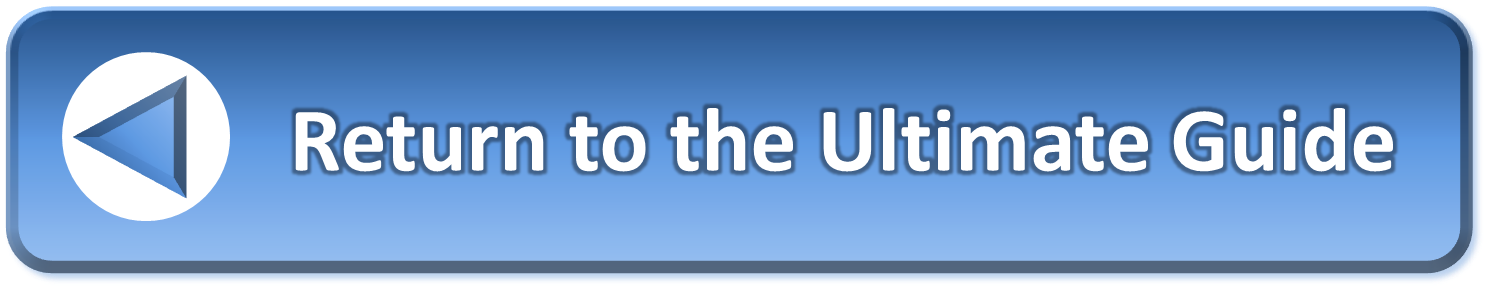Not a member yet?# From Where

### Preliminary list of standard 'From Where' questions.

• Where did the problem come from?
• What prompted your need to solve this problem now?
• When did you become aware of the problem?
• What are the origins of the problem?
• What is the source of the problem?
• What is the place where the problem came from?
• What is the root cause of the problem?
• Is there a single cause or are there many?
• What caused the problem? Doing something wrong or failing to do something?
• What are the reasons for the problem?
• What created the problem?
• Who created the problem?
• What added to the problem?
• Who added to the problem?
• How did you get to this place?
• How did the problem originate?
• How did the problem get to its current state?
• How did it develop?
• Did the problem originate through the normal course of events or was there some kind of trigger event?
• What path or trajectory has the problem been on?
• How did the problem get from the place where it originated to here?
• Why hasn’t it been dealt with?
• What have you done before?
• What actions have you already taken that failed to solve the problem?
• How many different ways have you tried to solve the problem?
• How have previous attempts to solve this problem failed?
• What’s holding you back?